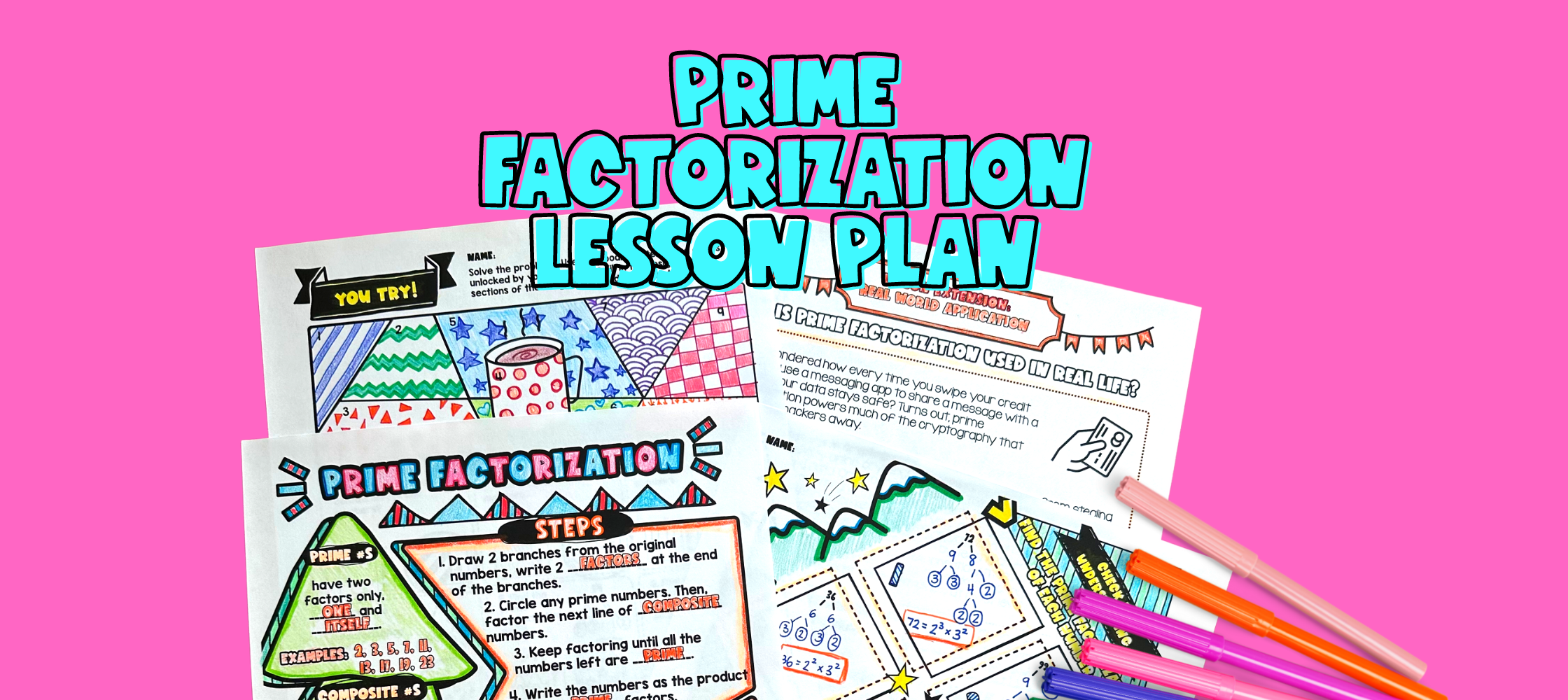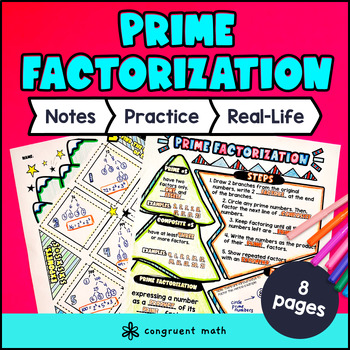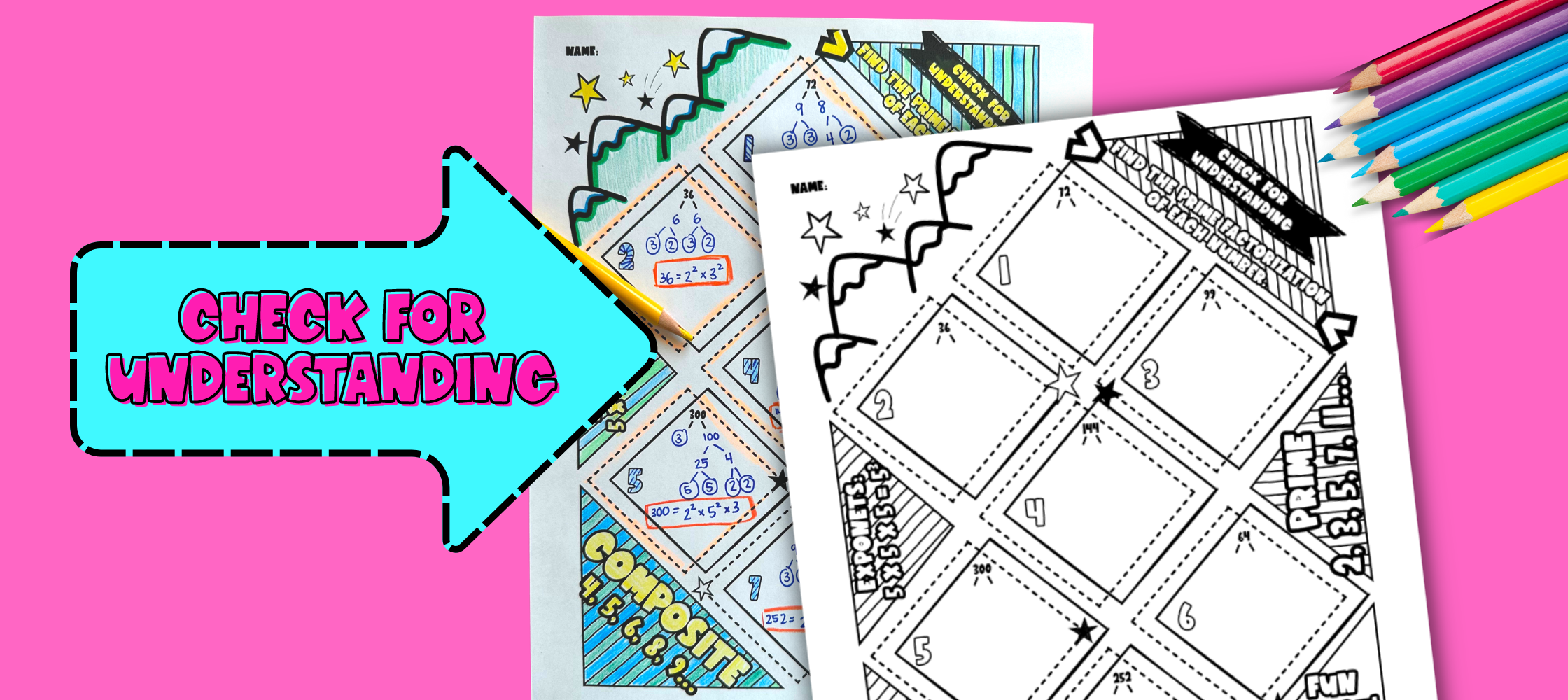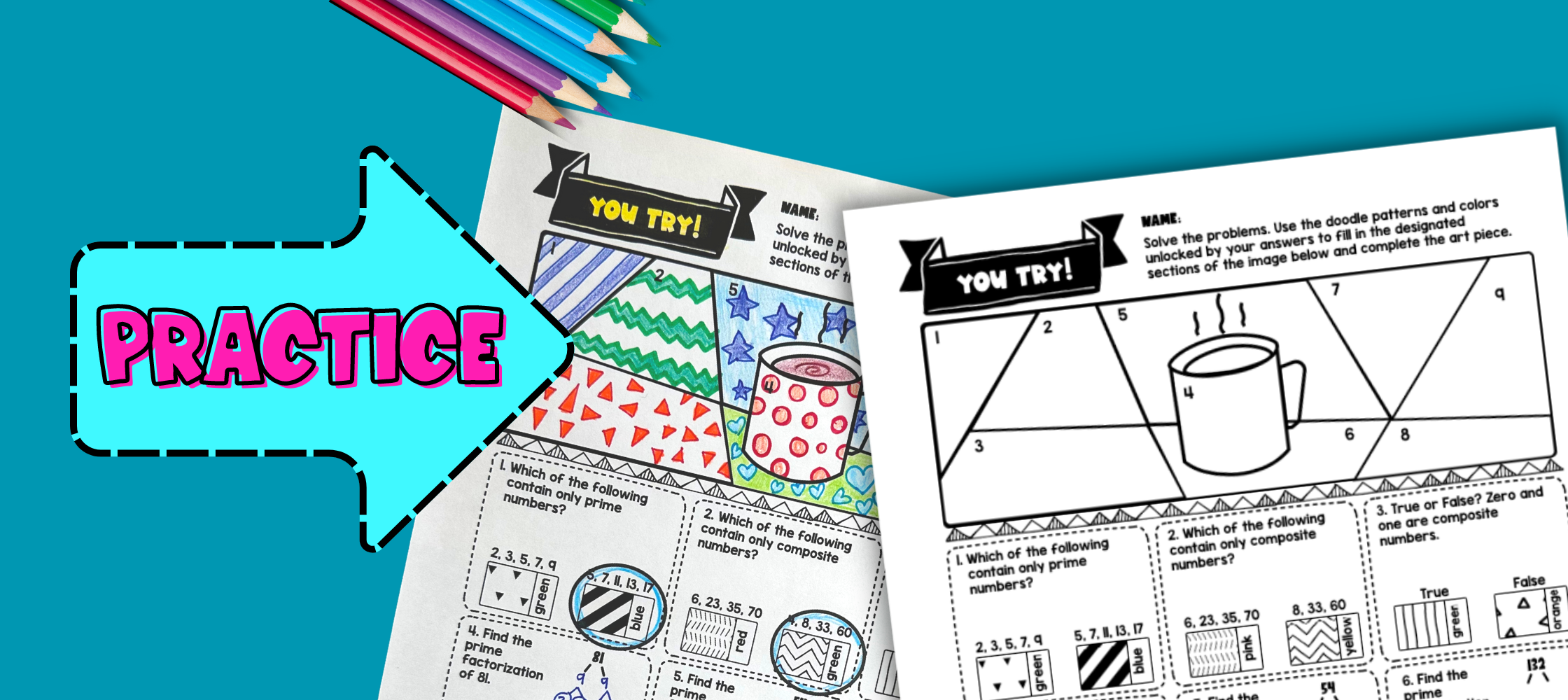# Prime Factorization Lesson PlanPin This

## Overview

Ever wondered how to teach prime factorization to 4th and 5th graders?

Use this artistic, real-life lesson plan to teach your students about prime factorization. Students will learn material with artistic guided notes (like sketch notes), practice and check for understanding with a doodle and color by number activity, and a maze worksheet.

The lesson culminates with a real-life application of how prime factorization powers the cryptography that keeps your data safe every time you swipe your credit card, buy something online, or tell a secret with a messaging app.

## Get the Lesson Materials\$3.99

## Learning Objectives

After this lesson, students will be able to:

• Differentiate between prime numbers and composite numbers

• Use factor trees to decompose a composite number into the products of its prime factors

• Represent prime factorization using exponents (i.e. 125 = 5^3)

• Describe how prime factorization is used in the real world for cryptography

Note: This lesson plan only includes practice with 2 and 3 digit numbers. See extensions for practice with longer numbers.

## Prerequisites

Before this lesson, students should be familiar with:

• Basic multiplication and division skills

• Understanding of the concepts of factors and multiples

• Basic understanding of fractions and decimals (optional, but helpful)

## Key Vocabulary

• Prime factorization

• Composite numbers

• Prime numbers

• Least common denominator

• Cryptography

## Procedure

### IntroductionPin This
• As a hook, ask students how computers keep them safe from hackers when they swipe their credit card or send a message on their phone. It all has to do with prime factorization! Refer to the last page of the guided notes as well as the FAQs below for ideas.

• Use the guided notes to introduce the concept of prime numbers, composite numbers, and prime factorization itself. Refer to the FAQ below for a walk through on this, as well as ideas on how to respond to common student questions.

• Introduce the steps to factor composite numbers. Then show students how to compute the prime factorization of 120 using a factor tree (120 = 2 x 2 x 2 x 3 x 5).

• Lastly, explain how 120 = 2 x 2 x 2 x 3 x 5 can be represented in exponent form as 120 = 2^3 x 3 x 5.

### Check for UnderstandingPin This
• Have students work through the problems on the check for understanding page, either collaboratively or independently.

• Walk around and spot-check student answers on the check for understanding activity.

• Based on student responses, reteach concepts that students need extra help with. If your class has a wide range of proficiency levels, you can pull out students for reteaching, and have more advanced students begin work on the practice exercises.

### PracticePin This
• Have students practice prime factorization using the maze activity. Walk around to answer student questions.

• Fast finishers can dive into the Doodle Math activity. You can assign it as homework for the remainder of the class.

### Real-World ApplicationPin This
• Bring the class back together, and introduce the concept of cryptography.

• Have students wrap class by writing down writing down ways they might use prime factorization in everyday life.

## Extensions

### Additional practice with up to 4 digit numbers

A fun, no-prep way to practice prime factorization is our Prime Factorization with Exponents Doodle & Color by Number activity (sold separately) — it's a fresh take on color by number or color by code. It includes three levels that help students practice finding the factors of 2-, 3- and 4-digit numbers. Each answer unlocks patterns that they can doodle onto an image.

There's also a special Earth Day version of the activity (sold separately), perfect for spring.

## Want more ideas and freebies?

Get my free resource library with digital & print activities—plus tips over email.